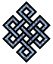#fail2ban bad ip database: ip 104.40.220.5

### | ip database | live view | stats | report | help | api key:

 ip: 104.40.220.5 hostname: 104.40.220.5 country: [??] UNKNOWN first reported: 14.10.2017 03:36.46 GMT+0200 last reported: 03.12.2018 12:38.13 GMT+0200 time period: 415d 10h 01m 27s total reports: 10 reported by: 2 host(s) filter(s): ssh (10) tor exit node no badips.com db Lookup## port scan of '104.40.220.5':

[-hide]
```# Nmap 6.40 scan initiated Sat Oct 14 03:37:02 2017 as: /usr/bin/nmap -sU -sS -O 104.40.220.5
Nmap scan report for 104.40.220.5
Host is up (0.0045s latency).
Not shown: 1000 open|filtered ports, 994 filtered ports
PORT     STATE  SERVICE
21/tcp   closed ftp
22/tcp   open   ssh
80/tcp   closed http
443/tcp  closed https
5900/tcp closed vnc
8010/tcp open   xmpp
No exact OS matches for host (If you know what OS is running on it, see http://nmap.org/submit/ ).
TCP/IP fingerprint:
OS:SCAN(V=6.40%E=4%D=10/14%OT=22%CT=21%CU=%PV=N%G=Y%TM=59E16A62%P=x86_64-pc
OS:-linux-gnu)SEQ(SP=FB%GCD=1%ISR=102%TI=Z%TS=A)OPS(O1=M5A0ST11NW7%O2=M5A0S
OS:T11NW7%O3=M5A0NNT11NW7%O4=M5A0ST11NW7%O5=M5A0ST11NW7%O6=M5A0ST11)WIN(W1=
OS:3890%W2=3890%W3=3890%W4=3890%W5=3890%W6=3890)ECN(R=Y%DF=Y%TG=40%W=3908%O
OS:=M5A0NNSNW7%CC=Y%Q=)T1(R=Y%DF=Y%TG=40%S=O%A=S+%F=AS%RD=0%Q=)T2(R=N)T3(R=
OS:N)T4(R=N)T5(R=Y%DF=Y%TG=40%W=0%S=Z%A=S+%F=AR%O=%RD=0%Q=)T6(R=N)T7(R=N)U1
OS:(R=N)IE(R=N)

OS detection performed. Please report any incorrect results at http://nmap.org/submit/ .
# Nmap done at Sat Oct 14 03:37:38 2017 -- 1 IP address (1 host up) scanned in 36.56 seconds
```
```Σ = 126 | Δt = 0.0051441192626953s
```